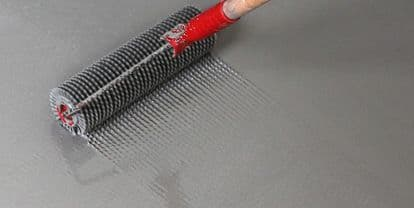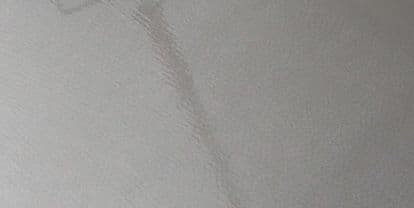# How much levelling compound do I need?

When it comes to knowing how much levelling compound you need? We've got you covered.5mm
HOW MUCH LEVELLING COMPOUND
When using Tiles

Generally, when using tiles this depth would be a minimum of 5mm.10mm
HOW MUCH LEVELLING COMPOUND
When Using Lino, LVT or Carpets

For sensitive floor covering, such as Lino, LVT (Luxury Vinyl Tile) or CARPETS, you would require a minimum of 10mm.

Please ensure the surfaces, the leveller is being applied to, are primed. If you're looking to source a primer, see our range of primers.

To correctly calculate the amount of levelling compound you require, use the follow formula or our product calculator.

HOW MUCH LEVELLING COMPOUND FORMULA
1.66kg x m2 x bed depth = total Kg divided by 20kg bags = QTY
1.66kg x 10m2 x 10mm = 166kg / 20 = 9 bags
Levelling Compound Calculator
m2
mm